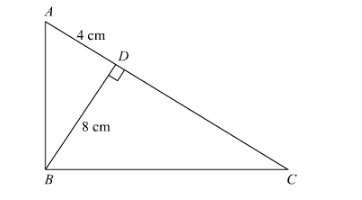# In the given figure, ∠ABC = 90° and BD ⊥ AC. If BD = 8 cm and AD = 4 cm, find CD.

Question:

In the given figure, $\angle \mathrm{ABC}=90^{\circ}$ and $\mathrm{BD} \perp \mathrm{AC}$. If $\mathrm{BD}=8 \mathrm{~cm}$ and $\mathrm{AD}=4 \mathrm{~cm}$, find $\mathrm{CD}$.Solution:

It is given that $\angle A B C=90^{\circ}$ and $B D \perp A C$.

When $B D=8 \mathrm{~cm}, A D=4 \mathrm{~cm}$ we have to find the $C D$.

Since $A B C$ is right angle triangle and $B D$ is perpendicular on $A C$, so

$\triangle D B A \sim \triangle D C B$ (AA similarity)

$\frac{B D}{C D}=\frac{A D}{B D}$

$\Rightarrow B D^{2}=A D \times D C$

$\Rightarrow(8 \mathrm{~cm})^{2}=4 \mathrm{~cm} \times D C$

$\Rightarrow D C=\frac{64 \mathrm{~cm}}{4 \mathrm{~cm}}=16 \mathrm{~cm}$

Hence, $C D=16 \mathrm{~cm}$